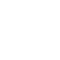# Wilson Hartnell Governor (Radial Spring Governor)

The spring controlled governor of the Wilson Hartnell type is shown in Fig. In this governor, the bell crank levers are pivoted at the ends of two arms which rotate with the spindle. The vertical arms of the bell crank lever support the balls and horizontal arms are attached to the sleeve through rollers. The two balls are connected by two main springs. The lower part of the sleeve is connected with an auxiliary spring through a lever. While rotating, when speed increases the ball move outwards and the sleeve moves upwards causing tension in both the springs.### Analysis of Wilson Hartnell Governor

m = Mass of each ball in kg,
M = Mass of the sleeve in kg,
P = Tension in the main spring in newtons,
S = Tension in the auxiliary spring in newtons,
sb = Stiffness of main spring in N/m,
sa = Stiffness of auxiliary spring in N/m,
FC = Centrifugal force of each ball in newtons,
r = Radius of rotation of balls in metres.
a,b = Lengths of the arms of the bell in metres.
x,y = Distance of hinge O1 for the lever from M and N respectively.

Upward force due to auxiliary spring at M = S $\frac{y}{x}$

Total downward force on the sleeve = Weight of the sleeve + S $\frac{y}{x}$

= M . g + S $\frac{y}{x}$

Taking moments about O, we get

$\left(\mathrm{F}_{\mathrm{C}}-\mathrm{P}\right) a=\frac{\mathrm{M} \cdot \mathrm{g}+\mathrm{S} \cdot\left(\frac{y}{x}\right)}{2} \times b$

Neglecting the obliquity of arms and moments due to weight of balls, and assuming suffices 1 and 2 for the minimum and maximum equilibriums respectively, we get as below:

∴ At minimum equilibrium speed, the equation (ii) can be written as

$\left(\mathrm{F}_{\mathrm{Cl}}-\mathrm{P}_{1}\right) a=\frac{\mathrm{M} \cdot \mathrm{g}+\mathrm{S}_{1} \times\left(\frac{y}{x}\right)}{2} \times b$

and at maximum equilibrium speed,

$\left(\mathrm{F}_{\mathrm{C} 2}-\mathrm{P}_{2}\right) a=\frac{\mathrm{M} \cdot \mathrm{g}+\mathrm{S}_{2} \times \frac{y}{x}}{2} \times b$

Subtracting equation

$\left[\left(F_{C 2}-F_{C l}\right)-\left(P_{2}-P_{1}\right)\right] a=\left(S_{2}-S_{1}\right) \frac{y}{x} \times\left(\frac{b}{2}\right)$

As r1 is increased to r2, the main springs are extended by an amount =2(r2 -r1) and the auxiliary spring is extended by an amount $=\left(r_{2}-r_{1}\right) \frac{b}{a}^{\frac{x}{x}}{x}$

$\mathrm{P}_{2}-\mathrm{P}_{1}=2 s_{b} \times 2\left(r_{2}-r_{1}\right)=4 s_{b}\left(r_{2}-r_{1}\right)$

and  $\mathrm{S}_{2}-\mathrm{S}_{1}=s_{a}\left(r_{2}-r_{1}\right)\left(\frac{b}{a}\right) \times\left(\frac{y}{x}\right)$

Substituting the values of (P2 -P1) and (S2 – S1) from equations (vi) and (vii) into equation (v), we get
$\begin{array}{l} {\left[\left(\mathrm{F}_{\mathrm{C} 2}-\mathrm{F}_{\mathrm{Cl}}\right)-4 s_{b}\left(r_{2}-r_{1}\right)\right] x=s_{a}\left(r_{2}-r_{1}\right)\left(\frac{b}{a}\right)\left(\frac{y}{x}\right)\left(\frac{y}{x}\right) \times\left(\frac{b}{2}\right)} \\ \left(\mathrm{F}_{\mathrm{C} 2}-\mathrm{F}_{\mathrm{C} 1}\right)-4 s_{b}\left(r_{2}-r_{1}\right)=\frac{s_{a}}{2}\left(r_{2}-r_{1}\right)\left[\frac{b}{a} \times \frac{y}{x}\right]^{2} \\ \therefore \quad 4 s_{b}+\frac{s_{a}}{2}\left[\frac{b}{a} \times \frac{y}{x}\right]^{2}=\frac{\mathrm{F}_{\mathrm{C} 2}-\mathrm{F}_{\mathrm{Cl}}}{r_{2}-r_{1}} \end{array}$
Note : When the auxiliary spring is not used, then Sa = 0.
$4 s_{b}=\frac{\mathrm{F}_{\mathrm{C} 2}-\mathrm{F}_{\mathrm{Cl}}}{r_{2}-r_{1}} \text { or } s_{b}=\frac{\mathrm{F}_{\mathrm{C} 2}- mathrm{F}_{\mathrm{Cl}}}{4\left(r_{2}-r_{1}\right)}$